## Thursday, November 18, 2010

### Jocelle Garcia's scribe posta) Maria walked 420m.

b) Walter walked 323m.

c) Maria, because she walked 97m further than Walter.a² = c² - b²
a² = 80cm² - 79cm²
a² = ( 80 x 80 ) - ( 79 x 79 )
a² = 6,400cm² - 6,241cm²
a² = 159cm²
√a² = √159cm²

### Jayvee's Pythagoras Scribe Post

5.Martin measured a rectangle and wrote: Width: 9 cm Length: 22 cm Diagonal: 23.8 cm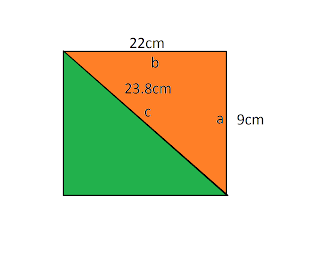a²+b²=c²
22²+9²=c²
(22x22)+(9x9)=c²
484cm2+81cm 2=565
√565cm²≠ 566.44
This can't form a rectangle because a and b does not equal c.

7.What is the height of the wheelchair ramp? Give your answer to the nearest tenth of a centimetre.

a²=c²-b²80²-79²=a²
6400-6241=159
159cm²=a²
√159cm=√a²
12.6cm²=a
The height of the wheelchair ramp is 12.6cm.

13.A cruise ship travels from Port Cassett north at a speed of 34 km/h for 2.5 h. Then it turns 90° and travels west at 30 km/h for 7.3 h. When it reaches Green Sea Island, how far is the ship from Port Cassett? Express your answer to the nearest kilometre.

a²+b²=c² 34x2.5=8.5
219²+8.5²=c² 30x7.3=219
(219x219)+(8.5x8.5)=c2
47961+72.25=48033.25
48033.25km=c²
√48033.25km=√c2
219.16km=c
The ship is 219.16km away from Port Cassett.

## Wednesday, November 17, 2010

### Carl's Homeworkbook Scibe Post

I have chosen to do the questions 4,7, and 8 in the homework book on page 33.
4. 6m2-1m2=h
36m-1m=35m
✓35m=5.9m

The ladder reaches 5.9m up the wall.

7. width=17cm
length=26cm
diagonal line=31cm
17cm2+26cm2=31cm2
289cm+676cm=961cm.

The quadrilateral is not a rectangle because 289cm +676cm does not equal 961cm, it equals 965.

8. 20 km/h for 10h west equals 200km
20 km/h for 7 1/2h south equals 150km
200km2 +150km2=?
40,000km + 22,500km= 62500km
✓62,500km =250km2

The ship is 250km away from the port.

### Nikki's Pythagoras Scribe Post

5. a) What is the area of each square attached to the legs of the right triangle?
answer The are for A (6cm) is 36 cm2. B (8cm) is 64 cm2.

b) What is the area for the square attached to the hypotenuse?
The answer is, The are for the hypotenuse is 100cm

c) What is the length of the hypotenuse?
The length for the hypotenuse is 10cm2.

8)The side view of a ramp at the Grocery store is in the shape of a right triangle. Determine the length of a ramp, to the nearest centimeter.

a2+b2=c2
502+2002 =c2
(50 times 50)+(200 times 200)=c2
2500+40000=c2
42500=c2
206=c

11)The right triangle below has a square attached to the hypotenuse. What the perimeter of the triangle. Give your answer to the nearest centimeter.

14) What are the length of b and c?Write your answer to the nearest tenth of a meter where appropriate.

The length for b is 4m. The length for c is 7.2.

## Tuesday, November 16, 2010

### Textbook work

#6 - Determine the length of the leg for each triangle.

6 a)-

c² - a² = b²
25² - 7² = b²
625 cm² - 49 cm² = b²
576 cm² = b²
√576 cm² = √b²
24 cm = b

6 b)-

t² - s² = r²
26² - 24² = r²
676 cm² - 576 cm² = r²
100 cm² = r²
√100 cm² = r²
10 = r

#9 -Tina wants to construct a path along the diagonal of her yard. What length will the path be? Express your answer to the nearest tenth of a metre.

a² + b² = c²
6² + 12² = c²
36 m² + 144 m² = c²
180 m² = c²
√180 m² = √c²
13.4 m = c

The length of the path will be 13.4 m long

#12-The hypotenuse of the triangle cuts the circle in half. What is the diameter of the circle? Express your answer to the nearest tenth of a centimetre.

a² + b² = c²
7² + 5² = c²
49 cm² + 25 cm² = c²
74 cm² = c²
√74 cm² = √c²
8.6 cm=c

The diameter of the circle is 8.6 cm

#15- The coordinate grid shown was drawn on centimetre grid paper. What is the length of line segment AB? Express your answer to the nearest tenth of a centimetre.

a² + b² = c²
4² +2² = c²
16 cm² +4 cm² = c²
20 cm² = c²
√20 cm² = √c²
4.5 cm = c

The line segment is 4.5 cm.

Homework!! - Pages 30 and 31 in the homework book

### Using the Pythagorean Relationship

Key Ideas Review.
Okay,so I'm doing odds,and I think Karen is doing evens.
Pg.30-31

1.The Pythagorean relationship can be used to determine the length of the hypotenuse of a right triangle when the lengths of the two legs are known. AGREE?

3.Determine the length of the hypotenuse.Show your work.
a)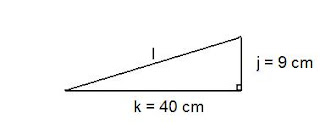k² + j² = l²
40
cm² + 9cm² = l²
(
40² x 40²) + (9² x 9²) = l²
1600
cm² + 81cm² = 1681cm²
1681cm² = √l²
41cm
²

b)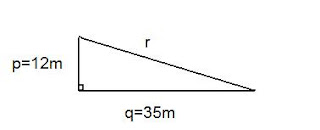p² + q² = r² 12m² + 35m² = r² (12² x 12² ) + (35² + 35²) = r²
144
+ 1225m² = 1369m²
1369 = r²
37cm²

5.Calculate the missing side length for each right triangle,to the nearest tenth of a centimeter.Show your work.
a)w² + x² = y²
w² + 25² = 36²
11 = 25 - 36
11 = 3.3
3.3²

b)f² + g² = h²
7cm
² + g² = 12cm²
49cm² + g² = 144cm²
144cm
² - 49cm² = 95cm²
95cm² = 9.7cm²
9.7cm
²

7.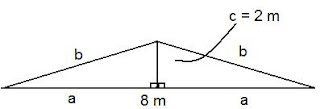A triangle is made up of two smaller congruent right triangles.

a)Find the Length of the hypotenuse for the right triangle,to the nearest tenth of a metre.Show your work.

4m
² + 2m² = b²
(4 x 4) + (2 x 2) = b
²
16m
² + 4m² = b²
20m² = 4.5m
b = 4.5m

b)Calculate the perimeter of the large triangle,to the nearest tenth of a metre.Show your work.
I don't know how to do this one..Help?

### Karen's Scribe Post

2. Use the relationship to determine the length of C in each triangle, to the nearest whole number. Show your work.
a)c² + d² = e²
c² + 24² = 26²
c² + 576 =676

c² + 576 - 576 = 676 - 576
c² = 100
c =
100
c = 10m

b)

b² + a² = c²
b² + 15² = 39²
b² + 225 = 1521
b² + 225 - 225 = 1521 - 225
b² = 1296
b =
√1296
b = 36cm

4. What is the length of each hypotenuse, to the nearest centimeter? Show you work.
a)
c² = a² + b²c² = 8² + 9²

c² = 64 + 81
c =
√145
c = 12cm (nearest centimeter)

b)
c² = a² + b²
c² = 6² + 10²
c² = 36 + 100
c =
136
c = 12cm

6. Find the height of a triangle with a base of 4 cm and a hypotenuse of 11 cm. Round to the nearest tenth of a centimeter. Show your w
ork.
a² + b² = c²a² + 4² = 11²
a² + 16 = 121
a² + 16 -16 = 121 - 16
a² = 105
a² =
105
a = 10.2cm

8. Ellie and Lucas are going the skateboard park to try out the new ramp. Is the new ramp a right triangle? Explain your thinking.
a² + b² = c²

2² + 3² = 5²4 + 9 = 13
13 ≠ 15 (5²)

No, it is not a right triangle because it doesn't equal the hypotenuse/5².

### Lizelle's ScribeQuestion 7

What is the missing length of the leg for each triangle? Give your answer to the nearest tenth of a millemetre.My formula is:

h= i-g=h
h=9-5=4
h=4What is the minimum distance the plaer at third base has to throw the ball to the runner out at first base? Express your answer to the nearest tenth.

I drew a picture, alike this one.
I labelled it, so when I was doing the formula
I wouldn't get confused.

a

a²+b²=c²
27²

## Monday, November 15, 2010

### Using The Phythagorean Relationship.

* How far does 2nd base throw to home base ?
The following picture shows the line from 2nd to home making a right triangle.a2+ b2= c2
(27x27) + (27x27) = c2
729m(squared) + 729m(squared) = c2
1458= c2
✔1458cm(squared)= ✔c2

38.18m= c

The following picture shows a right triangle. Find h/ c .a2 + b2= c2
(6x6) + (10x10) = c2
36cm(squared) + 100cm(squared)= c2

136cm2= c2
✔136cm(squared) =✔c2
11.66cm= c

The following picture shows a right triangle. You are only given r & t. Find s.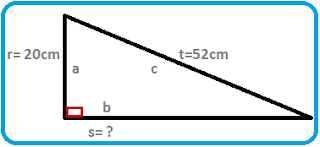a2 + b2 = c2
20(squared) + b2= 52(squared)
(20x20) + b2 = (52x52)
b2= 52(squared) - 20(squared)

b2= 2704- 400

b2= 2304 cm(squared)
✔ b2= ✔2304
b= 48cm

The following picture shows a right triangle. You are only given b and
c. Find a.a2 = c2 - b2
a2 = 9(squared) - 4(squared)
a2= 81cm2 - 16cm2

a2= 65cm
✔a2= ✔65cm
a= 8.06 cm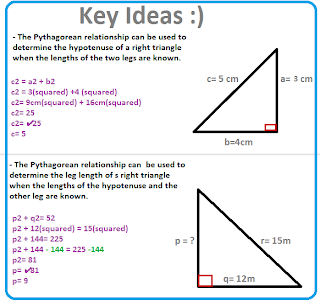HOMEWORK :)
Page 103 and 104 .

Questions 1, 2, 3 and 4.

Question 1:
Jack must determine the side length of a right triangle. He decides to draw it, and then measure it, as shown. Do you agree with the method that Jack is using? Explain .I agree with the method Jack is using, because once he knows what the two side lengths are, he can find the hypotenuse.

Question 2:

Kira calculated the missing side length of the right triangle.Is Kira correct ? If she is correct, explain how you know. If she is incorrect, explain the correct method.
Kira is not correct, because she forgot to do this part:
194cm(squared) = y2
✔194= ✔c2
13.9= c

## Tuesday, November 9, 2010

### Nicholas's Scribe Post.

K so,uhm,I chose to do question 7-8 for pages 90-91.

7.11 x 11 = 121 12 x 12 = 144
122-143

8.2 x 2 = 4 3 x 3 = 9
5-8

### Filimon Scribe Post

Home work: 98-100

9 pg 99- 17-18-19-20-21-2-23-24

15 pg 100- Arrange these number to least to greatest square- root of 27 - square root of 46- 5.8- 6.3- 7

If anyone can tell me how to make the square root sign please tell me in the comment box
below. Thanks!

### Ishaka's square roots scribe post

4) Estimate the square root of each number, to one decimal place. Check with a calculator.

a) 72
square root 64 =8 x 8
square root 72 = 8.__x 8.__
square root 81 = 9 x 9

I estimated that 8.49 was the square root of 72 calculator 8.48

b) 103
square root 100 = 10 x 10
square root 103 = 10.__ x 10.__
square root 121 = 11 x 11

I estimated that 10.15 x 10.15 was the square root of 103 calculator 10.14

c) 55
square root 49 = 7 x 7
square root 55 = 7.__ x 7.__
square root 64 = 8 x 8

I estimated that 7.42 x 7.42 was the square root of 55 calculator 7.41

6) What is an example of a whole number that has a square root between 9 and 10?

9 10
81 100

examples: 82, 83, 84, 85, 86, 87, 88, 89, 90, 91, 92, 93, 94, 95, 96, 97, 98, 99.

### Victoria's Square Root Post

Stella is planning an outdoor wedding. She would like a square dance ﬂoor with an area of 115 m2.

a) Determine the side length of the dance ﬂoor, to the nearest tenth of a metre.

b) Stella ﬁnds out that the dance ﬂoor will be made up of ﬂoorboards that each measure 1 m2. What are the two side lengths the dance ﬂoor can have that are closest to what she wants?

c) What are the two square areas for the dance ﬂoor that Stella can choose from?

d) Which area will Stella choose? Explain.

a.)10.7m b.) Sorry i dont know this question c.)100m2 or 121m2 d.) She will chose the 121m2 dance floor because its closer to the size she wanted.

New Question !

14. Alex is thinking of a number.

a) What number could he be thinking of?

b) Is there more than one answer? Explain

a.) 60 b.) No, there is only one answer and the nember must be between 49 and 64. The only multiple number is 12m this is the range of 60.

### Richards Scribe Post

Pages 98-100

16.a) 27m squared b) i cant find the answer . can you try finding the answer by commenting.

17.a) 324cm squared b)1296cm squared c) 36cm by 36cm squared

### Kevin's Square Root Scribe Post

Show you know:
a) Identify a whole number with a square root between 8 and 9.
b) How many whole numbers can you find that have a square root between 8 and 9? show you work.

a) First I changed the number 8 to 64 (8x8) and 9 to 81 (9x9).

64 ? 81

64 73 81

b) There are 16 whole numbers I found.

65 = 8.0

66 = 8.12

67 = 8.18

68 = 8.24

69 = 8.30

70 = 8.36

71 = 8.42

72 = 8.48

73 = 8.54

74 = 8.60

75 = 8.66

76 = 8.71

77 = 8.77

78 = 8.83

79 = 8.88

80 = 8.94

I used perfect squares to find these.

P.s I'm not sure if I'm right so can you guys help me out? Just post your answers at the comment section and I'll take a look at them.

### Nikki's Math Post

5.) Estimate each value, to one decimal place. Check your answer with a calculator.

a) 14 b) 86 c) 136 answer a) 3.7 b) 9.3 c) 11.7

19.) Estimate 160 100. Explain how you determined your estimate.
I think that 160 100 has a square root of 400 because 4 is the square root of 16 so all you
have to do is add the zeros.

Here is a picture to show you what square roots are :)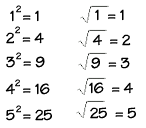Sorry I couldn't get the square root symbol.
Maybe you could help me and show me how to figure it out.

## Monday, November 8, 2010

### Victoria's Pythagoras Post

2.) Side Lengths : 7cm, 11cm, 15cm7.)Side Lengths : 9cm, 12cm, 15cmI did it wrong so here are the right answers :
A: Yes it is a right triangle
B: No it is not a right triangle
C: Yes it is a right triangle
D: Yes it is a right triangle
E: Yes it is a right triangle

18.) I cant figure it out so maybe you could help me out and show me how to do it ? :)

### Justin's Square Root Scribe Post

Show you know:
For each number of the following, use perfect squares to estimate the square root to one decimal place.Check your answers with a calculator

a)
18 b) ✔23 c) ✔35

You can estimate using perfect squares to find the square root to one decimal place like this:

✔18 is in between the perfect numbers 16 and 25. ✔16=4x4 ✔18=4.__ ✔25=5x5
✔18=4.__ because if you multiply 4.__ it equals 18.

✔23 is in between the perfect numbers 16 and 25. ✔16=4x4 ✔23=4.__ ✔25=5x5
23=4.__ because if you multiply 4.__ it equals 23.

✔35 is in between the perfect numbers 25 and 36. ✔25=5x5 ✔35=5.__ ✔36=6x6
35=5.__ because if you multiply 5.__ it equals 35.

## Sunday, November 7, 2010

### Paulo's Pythagoras Scribe Post

7) The sides of a right triangle measure 9 cm, 12 cm, and 15 cm

a) What is the area of each square attach to the three sides of the right triangle?
81 cm2 and 144cm2
b) Write an addition statement showing the relationship between the areas of the three squares?
81+144=225
c) Describe, using words and symbols, the relationship between side lengths of each square?

14) Show weather each triangle in the table is a right triangle.

Triangle
Side Lengths (cm)

A 9,12,15

B 7,8,11

C 7,24,25

D 16,30,34

E 10,11,14

A is a right triangle :9 square + 12 square= 15 square

B is not a right triangle :7 square + 8 square= almost 11 square

C is a right triangle : 7 square + 24 square = 25 square

D is a right triangle : 16 square + 30 square= 34 square

E is a right triangle : 10 square + 11 square = almost 14 square

18) The diagram is made of two right triangles and five squares.

### Glenn's Pythagoras Scribe Post

4. What are the areas of the three squared shown?
a) 30x30= 900mm
b)50x50= 2500mm
c)40x40= 1600mm

9. question .. a) Calculate the areas of three squares.
4x4= 16
2x2= 4
3x3= 9
b) Is this triangle a right angle.explain.
This is not a right triangle because 2 squared + 3 squared doesn't equal 4 squared.
12. Use the Pythagoras relationship to find the unknown area of each square.
a) 52cm square b) 676 mm square c) 65cm square d) 24cm square
17. FELLOW CLASSMATES .. can you help me answer this question, on the comment bar .. PLEASE AND THANK YOU (:
SORRY GUYS .. my spacing is messed up .. I tried editing it but it was still like this ..

### Cathlene's Pythagoras Scribepost

5. A right triangle has a side lenght of 40 mm, 75 mm, and 85 mm.

a. Sketch the triangle. Draw a square on each side of the triangle.

b. What are the areas of the three squares?

c. Write an addtion statement with the areas of the three squares

10. A triangle has side lenght of 120 mm, 160 mm, and 200 mm. Is the triangle a right triangle? Explain your reasoning.

The triangle is a right angle because both the legs of the triangle is equal to the area of the hypotenuse.

Legs: Hypotenuse:

120 x 120 = 14400 200 x 200 = 40000

160 x 160 = 25600

14400 + 25600 = 40000

15. Construction workers have begun to dig a hole for a swimming pool. They want to check if the angle they have dug is 90 degrees. They measure the diagonal as shown to be 9.5 m. Is the angle 90 degrees? Explain your reasoning.

Answer: No the hole they dug is not 90 degrees.

a + b = c

6 squared + 8 squared = c

6 x 6 = 36

8 x 8 = 64

36 + 64 = 100

A right triangle has a square attached to each side. Two of the squares have areas of 10 cm and 15 cm. What are possible areas for the third square? Draw a sketch for each solution.

### Sam's Pythagras Scribe post

Answers to 3, 8, 11, 16.

3. Kendra is wrong because 'p' is the hypotenuse.

8. If the triangle shown in the text book is a right angle than hypotenuse should be 60cm² because 20cm²+40cm² =60cm² .

11. a² + b² = c²

(6x6) + (5x5) = 8x8

36cm² + 25cm² = 61cm²

61cm² = 64cm²

-Since 61cm² does not equal 64cm² it is not a right triangle.

16. a² + b² = c²

(12x12) + (20x20) = c²

144cm² + 400cm² = 544cm²

√544cm² = 23.32cm

-Baldeep can be sure his box can be square by measuring the hypotenuse.

### Alec's Pythagoras Scribe Post

4. e. 30x30= 900mm
g. 50x50= 2500mm
f. 40x40= 1600mm
The area of e. is 900 square millimeters
The area of g. is 2500 square millimeters
The area of f. is 1600 square millimeters

9. a) 4x4=16 square cm.
2x2= 4 square cm.
3x3=9 square cm.

b) This is not a right triangle because 2 squared + 3squared doesn't equal 4 squared

12. a) a. squared + b. squared = c. squared
20 + 32 = 52 square cm.
b) a. squared + b. squared = c. squared
100 + 576 = 676 square mm.
c) c. squared - b squared = a. squared
90 - 25 = 65 square cm.
When the hypotenuse is there but one of the leg is missing, I figure it out that when you subtract the one of the legs to the hypotenuse, you will get the missing leg.

d) a. squared + b. squared = c. squared
12 + 12 = 24 square cm.

17. a) a. squared + b. squared = c. squared
21 squared + 28 squared = c .squared
441 + 784 = 1225 square cm.
b) a. squared + b. squared = c. squared
12 squared + 5 squared = c.squared
144 + 25 = 169 square cm.

### Ryan's Pythagoras Scribepost

5. A right triangle has side lengths of 40mm, 75mm, and 85mm.
a) Sketch the triangle. Draw a square on each side of the triangle.
b) What are the areas of the three squares?
The area for square a, is 1,600mm2 (squared).
The area for square b, is 5,625mm2 (squared).
The area for square c, is 7,225mm2 (squared).
c) Write an addition statement with the areas of the three squares.
a(squared)+b(squared)=c(squared)
40(squared)+75(squared)=85(squared)
1,600(squared)+5,625(squared)=7,225(squared)
7,225mm(squared)=7,225mm(squared)
10. A triangle has side lengths of 120mm, 160mm, and 200mm. Is the triangle a right triangle? Explain your reasoning.
Yes, this is a right triangle because the sum of the area of each leg, is equal to the area of the hypotenuse.
a(squared)+b(squared)=c(squared)
120(squared)+160(squared)=200(squared)
14,400(squared)+25,600(squared)=40,000(squared)
40,000(squared)=40,000(squared)
15. Construction workers have begun to dig a hole for a swimming pool. They want to check that the angle they have dug is 90 degrees. They measure the diagonal as shown to be 9.5m. Is the angle 90 degrees? Explain your reasoning.

No, the angle is not 90 degrees because the sum of the area of each leg, is not equal to the area of the hypotenuse.
19. A right triangle has a square attached to each side. Two of the squares have areas of 10cm(squared) and 15cm(squared). What are the possible areas for the third square? Draw a sketch for each solution.
There is only one possible area for the third square, and that is the sum of the area of each leg: 25cm(squared). There is only one possible area because it is a right triangle.